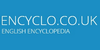pressure dropA slope or incline. In plant pathology, an observed progressive change with distance of the concentration of spores, lesions or diseased plants, or in the value of an environmental attribute.A vector of partial derivatives of a function that operates on vectors. Intuitively, the gradient represents the slope of a high-dimensional surface.
Found on http://www.encyclo.co.uk/local/20090(1) A measure of slope (soil- or water-surface) in meters of rise or fall per meter of horizontal distance. (2) More general, a change of a value per unit of distance, e.g. the GRADIENT in longshore transport causes EROSION or ACCRETION. (3) With reference to winds or currents, the rate of increase or decrease in speed, usually in the vertical; or ...
Found on https://www.encyclo.co.uk/local/20127[n] - a graded change in the magnitude of some physical quantity or dimension 2. [n] - the property possessed by a line or surface that departs from the horizontalAlso called slope. The gradient indicates how steeply a line slopes. You will come across this when using the linefitting technique. A line is described by its gradient and intercept, the point at which the line crosses the y-axis. See also Linefitting.
Found on http://www.encyclo.co.uk/local/20429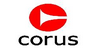The fall or rise per unit length of land, railway, road.
Found on http://www.corusconstruction.com/en/design_guidance/the_blue_book/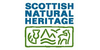Angle or slope of the ground or path; long gradient refers to the slope along the path line.
Found on http://www.encyclo.co.uk/local/20717Gra'di·ent adjective [ Latin gradiens , present participle of gradi to step, to go. See Grade .] 1. Moving by steps; walking; as, gradient automata. Wilkins. 2. Rising or descending by regular degrees of inclination; as, the gradient
Found on http://www.encyclo.co.uk/webster/G/48Gra'di·ent noun 1. The rate of regular or graded ascent or descent in a road; grade. 2. A part of a road which slopes upward or downward; a portion of a way not level; a grade. 3. The rate of increase or decrease of a variable magnitude, or the curve which represents it; as, a...
Found on http://www.encyclo.co.uk/webster/G/48<physics> Mathematical term for the operator which determines the magnitude and direction of the greatest rate-of-change of a given function with position. Similarly used to describe such a rate-of-change. ... For instance, at a given point on a hill, the slope of the hill in the steepest uphill direction is the gradient of the altitude funct...
Found on http://www.encyclo.co.uk/local/20973noun the property possessed by a line or surface that departs from the horizontal; `a five-degree gradient`
Found on https://www.encyclo.co.uk/local/20974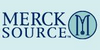(gra´de-әnt) rate of increase or decrease of a variable value, or its representative curve. concentration gradient a difference in the concentration of a substance on two sides of a permeable barrier. edge gradient in radiology, the penumbra or partia...
Found on http://www.encyclo.co.uk/local/21001• (a.) Adapted for walking, as the feet of certain birds. • (a.) Rising or descending by regular degrees of inclination; as, the gradient line of a railroad. • (n.) The rate of regular or graded ascent or descent in a road; grade. • (n.) A part of a road which slopes upward or downward; a portion of a way not level; a grade. &bu...(from the article `canals and inland waterways`) The geographic restriction is that, unlike roads, railways, or pipelines, which are adaptable to irregular natural features, waterways are confined ...
Found on http://www.britannica.com/eb/a-z/g/55(from the article `fluid mechanics`) The equation is written in a compact vector notation which many readers will find totally impenetrable, but a few words of explanation may help some ... The contours on a standard map are lines along which the height of the ground above sea level is constant. They usually take a complicated form, but...
Found on http://www.britannica.com/eb/a-z/g/55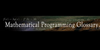Vector of first partial derivatives of a function (assumed to be differentiable at least once). Here this is denoted grad_f(x), where f is the function and x is a point in its domain.
Found on http://glossary.computing.society.informs.org/index.php?page=G.html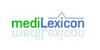Type: Term Pronunciation: grā′dē-ĕnt Definitions: 1. Rate of change of temperature, pressure, magnetic field, or other variable as a function of distance, time, or other continuously changing influence.
Found on http://www.medilexicon.com/medicaldictionary.php?t=38162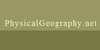The steepness of a slope as measured in degrees, percentage, or as a distance ratio (rise/run).
Found on http://www.physicalgeography.net/physgeoglos/g.html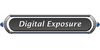A smooth spread between colours
Found on http://www.encyclo.co.uk/local/21048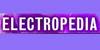vector quantity grad f associated at each point with a scalar field quantity f, having a direction normal to the surface on which the field quantity has a constant value, in the sense of increasing value of f, and a magnitude equal to the absolute value of the derivative of f with respect to distance in this normal direction NOTE 1 - The gradient e...
Found on http://www.electropedia.org/iev/iev.nsf/display?openform&ievref=101-11-37A vector of partial derivatives of a function that operates on vectors. Intuitively, the gradient represents the slope of a high-dimensional surface.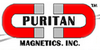Indicates the change in magnetic strength between points measured at different distances perpendicular to the magnetic field.
Found on http://www.puritanmagnetics.com/1/magnet/glossary_of_magnetic_terms.asp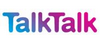On a graph, the slope of a straight or curved line. The slope of a curve at any given point is represented by the slope of the tangent at that pointIn mathematics, the gradient is a generalization of the usual concept of derivative of a function in one dimension to a function in several dimensions. If {math|f(x1, ..., xn)} is a differentiable, scalar-valued function of standard Cartesian coordinates in Euclidean space, its gradient is the vector whose components are the n partial derivatives ...